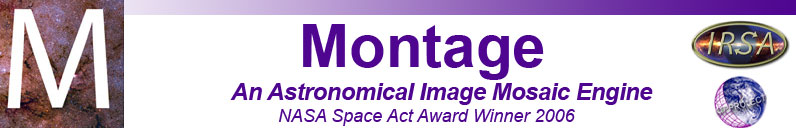# mRotate

Description:
Rotates a FITS image by an arbitrary angle. This module is meant for quick-look only; it is not flux conserving.
Syntax:
mRotate [-d level] [-s statusfile] [-r rotang] in.fits out.fits [ra dec xsize [ysize]]

Switches:

-d level
Print out additional debugging information (level can be 1-3)
-s statusfile
Output and errors are written to statusfile instead of stdout.
-r rotang
Provide an angle (in degrees) to rotate the image.

Arguments:

in.fits
Input FITS image.
out.fits
Path to output (rotated) FITS image.
ra dec xsize
Center location and width (in degrees) of output image - optional. By default, entire input image area will be included in output image.
ysize
Height (in degrees) of output image, if a new center location and width are provided. Optional - defaults to xsize.
Results:
[struct stat="OK"]
Output file will contain the same image as the input file, but rotated by rotang degrees. If a new center location and width were provided, the output image will be the "subimage" covering that area.

Examples:

\$ mRotate -r 90 in.fits out.fits
[struct stat="OK"]

Return Codes:

• [struct stat="OK"]
• [struct stat="ERROR", msg="Usage: mRotate [-d level] [-s statusfile] [-r rotang] in.fits out.fits [ra dec xsize [ysize]]"]
• [struct stat="ERROR", msg="No status file name given"]
• [struct stat="ERROR", msg="Cannot open status file: statusfile"]
• [struct stat="ERROR", msg="No rotation angle given"]
• [struct stat="ERROR", msg="Rotation angle string is invalid: 'rotang'"]
• [struct stat="ERROR", msg="No debug level given"]
• [struct stat="ERROR", msg="Debug level string is invalid: 'level'"]
• [struct stat="ERROR", msg="Debug level value cannot be negative"]
• [struct stat="ERROR", msg="Invalid input file 'in.fits'"]
• [struct stat="ERROR", msg="Invalid output file 'out.fits'"]
• [struct stat="ERROR", msg="Center RA string (ra) cannot be interpreted as a real number"]
• [struct stat="ERROR", msg="Center Dec string (dec) cannot be interpreted as a real number"]
• [struct stat="ERROR", msg="X size string (xsize) cannot be interpreted as a real number"]
• [struct stat="ERROR", msg="Y size string (ysize) cannot be interpreted as a real number"]
• [struct stat="ERROR", msg="Invalid 'x' size"]
• [struct stat="ERROR", msg="Invalid 'y' size"]
• [struct stat="ERROR", msg="Location is off image"]
• [struct stat="ERROR", msg="No pixels match output area"]
• [struct stat="ERROR", msg="No pixels match input area"]
• [struct stat="ERROR", msg="wcsinit() failed"]
• [struct stat="ERROR", msg="Image file in.fits missing or invalid FITS"]
• [struct stat="ERROR", status=flag, msg="FITS library error"]